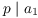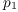# The Fundamental Theorem of Arithmetic

Theorem. (Fundamental Theorem of Arithmetic) Every integer greater than 1 can be written in the formIn this product,and the's are distinct primes. The factorization is unique, except possibly for the order of the factors.

For instance,I need a couple of lemmas in order to prove the uniqueness part of the Fundamental Theorem. In fact, these lemmas are useful in their own right.

Theorem. Ifand, then.

Proof. WriteThenNowand(since), so.Theorem. If p is prime and, thenfor some i.

For, the result says that if p is prime and, thenor. This is often called Euclid's lemma.

Proof. The result is trivial if, since it says that if, then.

Do the casefirst. Suppose, and suppose. I must show., and p is prime, soor. If, then, which contradicts. Therefore,. By the preceding theorem,. This establishes the result for.

Assume, and assume the result is true when p divides a product of with less than n factors. Suppose that. Grouping the terms, I haveBy the case, eitheror. If, I'm done. Otherwise, if, then p divides one of,, ...,, by induction. In either case, I've shown that p divides one of the's, which completes the induction step and the proof.Using these results, I'll prove the Fundamental Theorem of Arithmetic.

Proof. ( Fundamental Theorem of Arithmetic) First, I'll use induction to show that every integer greater than 1 can be expressed as a product of primes.is prime, so the result is true for.

Suppose, and assume every number less than n can be factored into a product of primes. If n is prime, I'm done. Otherwise, n is composite, so I can factor n as, where. By induction, a and b can be factored into primes. Thenshows that n can, too.

Now I'll prove the uniqueness part of the Fundamental Theorem.

Suppose thatHere the p's are distinct primes, the q's are distinct primes, and all the exponents are greater than or equal to 1. I want to show that, and that eachisfor some b --- that is,and.

Consider. It divides the left side, so it divides the right side. By the last lemma,for some i. Butis(times), so again by the last lemma,. Sinceandare prime,.

To avoid a mess, renumber the q's sobecomesand vice versa. Thus,, and the equation readsIf, cancelfrom both sides, leavingThis is impossible, since nowdivides the left side, but not the right.

For the same reasonis impossible.

It follows that. So I can cancel the's off both sides, leavingKeep going. At each stage, I pair up a power of a p with a power of a q, and the preceding argument shows the powers are equal. I can't wind up with any primes left over at the end, or else I'd have a product of primes equal to 1. So everything must have paired up, and the original factorizations were the same (except possibly for the order of the factors).Recall that the least common multiple of nonzero integers a and b is the smallest positive integer divisible by both a and b. The least common multiple of a and b is denoted.

For example,Here's an interesting fact that is easy to derive from the Fundamental Theorem.

Proposition. If a and b are nonzero integers, then.

Proof. (sketch) Factor a and b in products of primes, but write out all the powers (e.g. writeas):Here the q's are the primes a and b have in common, and the p's and r don't overlap. Picture:From the picture,Thus,.You might see if you can prove this proposition without using the Fundamental Theorem (that is, without factoring a and b into products of primes.

Here's how this result looks for 36 and 90:We haveand; notice that.

Contact information

Copyright 2019 by Bruce Ikenaga